+

# Literal Equations

##### Rating:
(4)
• (1)
• (0)
• (1)
• (1)
• (1)
Author: Sophia Tutorial
##### Description:

Use inverse operations to rewrite a literal equation.

(more)
Tutorial
what's covered
1. Definition of Literal Equation
2. Formulas as Literal Equations
3. Rewriting Literal Equations

# 1. Definition of Literal Equation

A literal equation is an equation that has more than one variable. In math, we work with literal equations all the time. For example, the slope–intercept form of a line is a literal equation: y = mx+b. This is because it has more than one variable.

term to know
Literal equation
an equation with more than one variable

# 2. Formulas as Literal Equations

Formulas are common literal equations. Formulas relate variables together. For example, we can use a formula to relate the length and width of a rectangle to its area. We can rewrite formulas to create expressions for other variables in the equation. For example: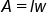Formula for the area of a rectangle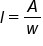Divide by w; expression for length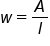Divide by l, expression for width

# 3. Rewriting Literal Equations

We can rewrite literal equations to express other variables by apply inverse operations. More specifically, we look at what operations are being applied to the variable we wish to isolate, as well as in what order they are being applied. To isolate the variable, we apply the inverse operations in reverse order. This is shown below with several common formulas: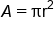Formula for the area of a circle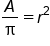Divide both sides by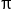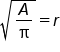Take square root of both sides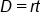Formula for distance, rate, and time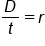Divide both sides by tFormula for distance, rate, and timeDivide both sides by r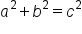Pythagorean Theorem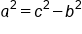Subtract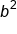from both sides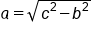Take square root of both sidesPythagorean Theorem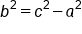Subtract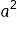from both sides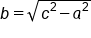Take square root of both sides

summary
The definition of literal equations are equations that have more than one variable. Formulas are literal equations and are used often in mathematics. Depending on what kind of information you are given, you may wish to rewrite literal equations, or express the equations and formulas in different ways.

Terms to Know
Literal Equation

an equation with more than one variable

Rating Header